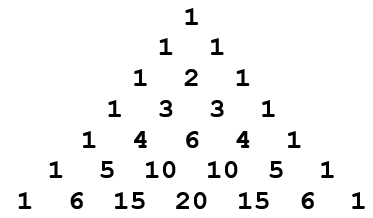New update is available. Click here to update.
Last Updated: 9 Jan, 2021
##### Find Kth row of Pascal's Triangle
Easy
Problem statement#### In Mathematics, Pascal's triangle is a triangular array where each entry of a line is a value of a binomial coefficient. An example of Pascal’s triangle is given below.#### Example :-

``````INPUT : K = 2
OUTPUT: 1 1

In the above example, K = 2, Hence the 2nd row from the top of pascal’s triangle, as shown in the above example is 1 1.

INPUT   : K = 4
OUTPUT  : 1 4 6 4 1

In the above example, K = 4, Hence the 4th row from the top of pascal’s triangle, as shown in the above example is 1 3 3 1.
``````
##### Input Format
``````The first line of input contains an integer 'T' representing the number of the test case. Then the test case follows.

The first and the only line of each test case contains a single integer “K”.
``````
##### Output Format:
``````For every test case, print a single line containing 'R' space-separated integers showing the Kth row of pascal’s triangle, where 'R' is the number of elements in a particular row.

The output of each test case will be printed in a separate line.
``````
##### Note
``````You don’t have to print anything, it has already been taken care of. Just implement the given function.
``````
##### Constraints:
``````1 <= T <= 50
1 <= K <= 50

Where ‘T’ is the number of test cases, ‘K’ is the input row number.

Time limit: 1 sec.
``````Approaches

## 01ApproachIn this approach, we find the row elements of the previous row using recursion, and based on the values of previous row elements, we will evaluate the current row elements.

1. We are given a function kthRow() which takes an integer ‘K’ as the only parameter and returns an integer vector. This is the definition of our recursive function too.
2. As the base condition of our recursive function, we will check if ‘K’ is equal to 1 or not. If ‘K’ is 1, we will return the vector containing 1.
3. Now we will call our recursive function kthRow() with K - 1 as our new parameter to store the previous row elements.
4. Now by adding the previous row elements, we will find the current row elements and then return the current row.